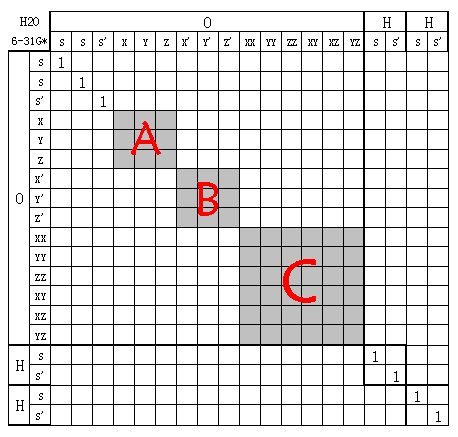# 由Mulliken布居和Lowdin布居再谈旋转不变性问题

## 2. 原子基函数的正交性

STO(Slater type orbitals)：相对于类氢原子轨道修改了径向部分的形式，使其波节消失，每个STO仍对应于一个原子轨道（虽然高精度计算时也可以用多个STO展开一个原子轨道）。不同类型（指s、p、d...后同）之间的轨道都正交；相同类型不同主量子数轨道间不都正交，不正交情况例如3px与4px。

## 3. 旋转分子引起的基函数的变换## 4. Mulliken布居、Lowdin布居与的旋转不变性问题

Mulliken布居可以写成矩阵形式，P为密度矩阵，S为重叠矩阵，则PS矩阵的第(i,i)矩阵元就是第i个基函数的Gross原子布居数，也就是这个基函数被Mulliken布居分配到的电子数，显然∑[k∈α](PS)(k,k)就是α原子的Mulliken电荷。Lowdin布居就是做Mulliken布居之前先用Lowdin正交化方法使全部基函数之间正交化，变换矩阵为X=S^(-0.5)。设P'、C'、S'为正交化后基函数的密度矩阵、系数矩阵（只含占据轨道的展开系数，矩阵元i,j为第j个分子轨道第i个基函数系数）和重叠矩阵，则P'S'=P'=C'C'^(T)=X^(-1)CC^(T)X^(-1)^T=S^(0.5)CC^(T)S^(0.5)^T=S^(0.5)PS^(0.5)，所以S^(0.5)PS^(0.5)的第(i,i)个矩阵元就是Lowdin布居给基函数i分配的电荷。注意由于AM1、PM3等半经验方法对重叠矩阵近似为单位矩阵，此时Lowdin布居与Mulliken布居是等价的。

S'=X`SX。当X为酉矩阵时，S'^(0.5)=X`S^(0.5)X，证明见CPL,393,p209-212。
P'=X^(-1)PX`^(-1)。当X为酉矩阵时，可进一步写为X`PX。

Mulliken布居所得α原子电荷Q(α)=∑[k∈α](PS)(k,k)=∑[k∈α](X^(-1)PX`^(-1)X`SX)(k,k)=∑[k∈α](X^(-1)PSX)(k,k)。

Q(α)=∑[k∈α](S'^(0.5)P'S'^(0.5))(k,k)=∑[k∈α](X`S^(0.5)XX`PXX`S^(0.5)X)(k,k)=∑[k∈α](X`S^(0.5)PS^(0.5)X)(k,k)，然后如同证明Mulliken布居的过程一样，利用X可视为原子对角块矩阵特点，可写为∑[k∈α](S^(0.5)PS^(0.5))(k,k)。所以，旋转不会令Lowdin布居所得原子电荷改变。